# The Retired Profesor simplifies Algebra: Combining Polynomials

## This of a free resource where insights into algebra are provided to help both students and those helping students with algebra.

Combining polynomial expressions is easy once polynomials are thought of as analogous to numbers. One thing that makes things understandable is to use something familiar, not start from nothing and attempt to learn without using prior skills.

In the case of polynomials, the four arithmetic operations of addition, subtraction, multiplication, and division are the same as those same operations are for numbers.

This material follows exponents since polynomials use exponents, and that material is necessary before we reverse the polynomial process in factoring, a future segment.

### Operating on Polynomials

Arithmetic operations can be applied to polynomials much like they are applied to numbers.

It is best to think of numbers in expanded form, then see how they match polynomials.

If we express the number 435 in expanded form it looks like

4(100) + 3(10) + 5, or 4(102) + 3(10) + 5

Compare the above with 4x2 + 3x + 5

Now add 4x2 + 3x + 5 to 2x2 + 6x + 2.

This is like adding 435 to 262.

435

+262

697

(4x2 + 3x + 5) + (2x2 + 6x + 2) = 6x2 + 9x + 7, obtained by adding like terms from each polynomial.

So, do not panic, you use the same techniques you learned for arithmetic.

Actually, polynomial addition is easier, since there is no need to carry.

The numbers are not restricted to non-negative integers, negative values and fractions are allowed.

Subtraction is also the same technique as the one you know.

628 – 312 = 316.

Subtract 3x2 + x + 2 from 6x2 + 2x + 8

(6x2 + 2x + 8) – (3x2 + x + 2) = 3x2 + x + 6

Notice we subtract like terms from like terms.

Now multiply.

321 X 12

321

12

642

321

3852

Why is the 321 indented from the right?  Well, look at how it occurs.  1(10) X 1 = 1(10), so the 1 belongs in the 10 column.  1(10) X 2(10) = 2(102), so the 2 belongs in the 102 column.  And 1(10) X 3(102) = 3(103), so the 3 belongs in the 103 column.

Notice that 3(10) will not multiply by anything to get a value in the units column.

Notice each digit from one number multiplied by each digit from the other number.

(3x2 + 2x + 1)( x + 2) = 3x3 + 6x2 + 2x2 + 4x + x + 2

= 3x3 + 8x2 + 5x + 2

Now divide (4x2 + 6x + 5) ÷ (2x + 1)

If you divide 465/21 you get 21 with a remainder of 2, or 22 + 3/21.

Divide (4x2 + 7x + 5) ÷ (2x + 2) = 2x + 2 + 3/(2x + 1)

2 x + 2

2x + 1)4x2 + 6x + 5

4x2 + 2x

4x + 5

4x + 2

3

So, what is done with polynomials is the same thing done with arithmetic.  It does allow negative numbers, fractions, and larger numbers, but the technique is the same.

Unfortunately, many students become calculator dependent, so these are new techniques to them.  But to those who learned these techniques at an early age, the operations with polynomials are the same as those with which they are familiar.

### Other Topics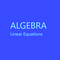Algebra simplified into a brief but thorough free resource can benefit both a parent helping a student and the student. This segment addresses linear equations.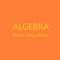This is the second part of a free resource to help with the basic understqanding of algebra. It can be used by students and those helping students witht the understanding.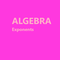This is a free resource on understanding algebra, and presented in a short presentation aimed at making things clear. It may help thoses studying algebra and those trying to help.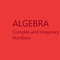This is a free to use algebra resource. It is designed to make it easy to understand complex and imaginary numbers for students and those assisting students with learning.

This article contains links to affiliate programs and Adsense advertising.  These must use cookies to allow for proper crediting, and allow me to earn from qualifying purchases. As an Amazon Associate I earn from qualifying purchases.

Updated: 03/01/2021, blackspanielgallery
1

Only logged-in users are allowed to comment. Login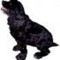blackspanielgallery on 02/12/2021

I fixed it. I had changed the number so we did not get a value past 10 when adding, and left the wording.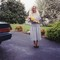DerdriuMarriner on 02/12/2021

blackspanielgallery, Thank you for the practicalities and products.
I appreciate the explanations, since I always loved polynomials in school.
Under the multiplied number example, you ask us, "Why is the 963 indented?" I do not see that number anywhere.

#### You might also like

Learn Algebra and Trigonometry with Rap Videos

Learn the hard to grasp Algebra and Trigonometry basics from inspiring math t...

The Retired Professor Simplifies Algebra: Systems of Equations

Insights into algebra with attention to systems of linear equations is the fo...

Disclosure: This page generates income for authors based on affiliate relationships with our partners, including Amazon, Google and others.